# 用Python爬虫抓取免费代理IP--  Illustrations by Ash Thorp & Maciej Kuciara --

blog地址：zhihu.com/people/hdmi-blog

\

Python版本：Python3.6

IDE: Sublime Text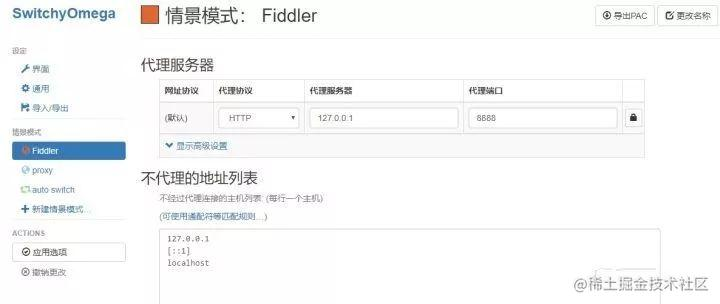``复制代码``
1. `import requests`
2. ``
3. `proxy = '127.0.0.1:8888'`
4. `proxies = {`
5. `   'http':'http://' + proxy,`
6. `   'https':'http://' + proxy`
7. `}`
8. ``
9. `try:`
10. `   response = requests.get('http://httpbin.org/get',proxies=proxies)`
11. `   print(response.text)`
12. `except requests.exceptions.ConnectionError as e:`
13. `   print('Error',e.args)`

``复制代码``
1. `{`
2. `   "args":{}，`
3. `   "headers":{`
4. `       "Accept":"*/*",`
5. `       "Accept-Encoding":"gzip, deflate",`
6. `       "Connection":"close",`
7. `       "Host":"httpbin.org",`
8. `       "User-Agent":"python-requests/2.18.4"`
9. `   },`
10. `   "origin":"xx.xxx.xxx.xxx",`
11. `   "url":"http://httpbin.org/get"`
12. `}`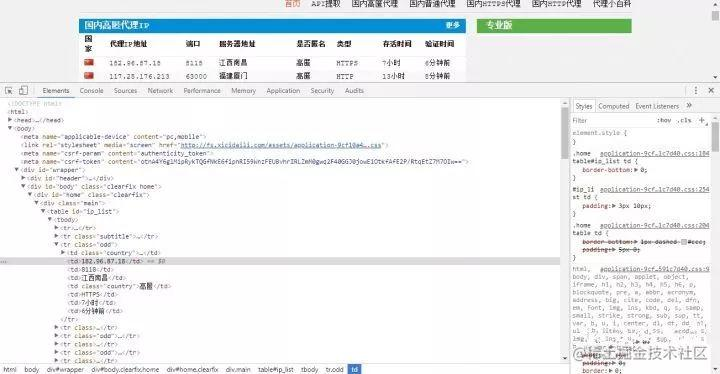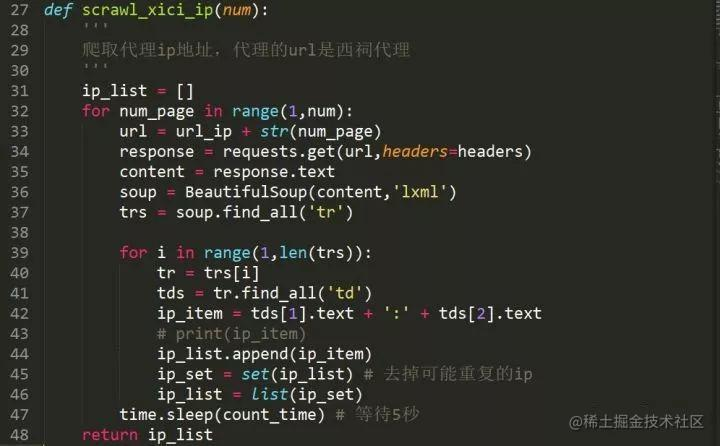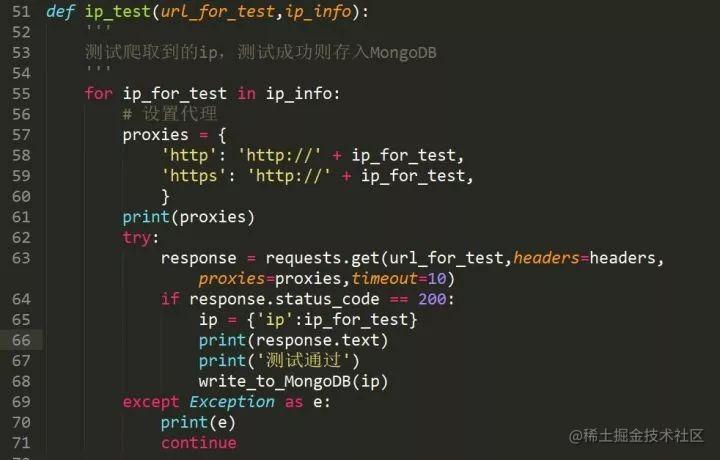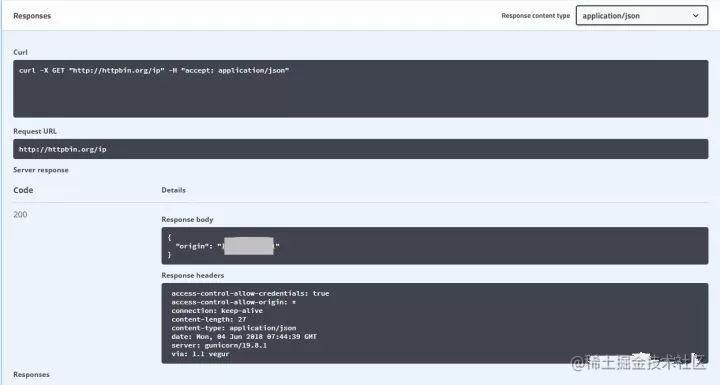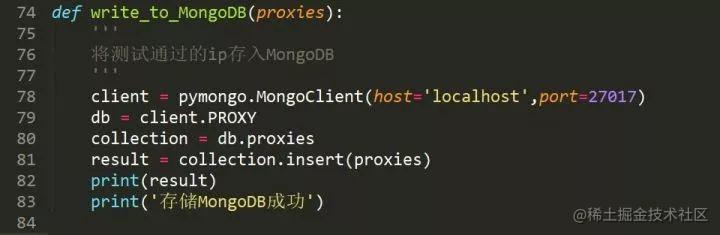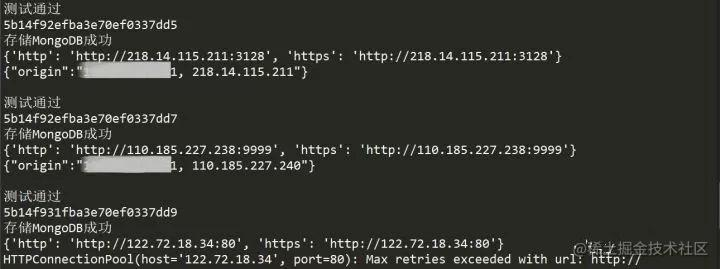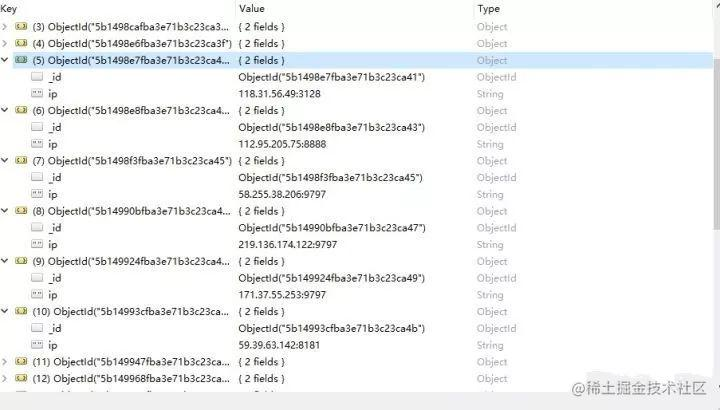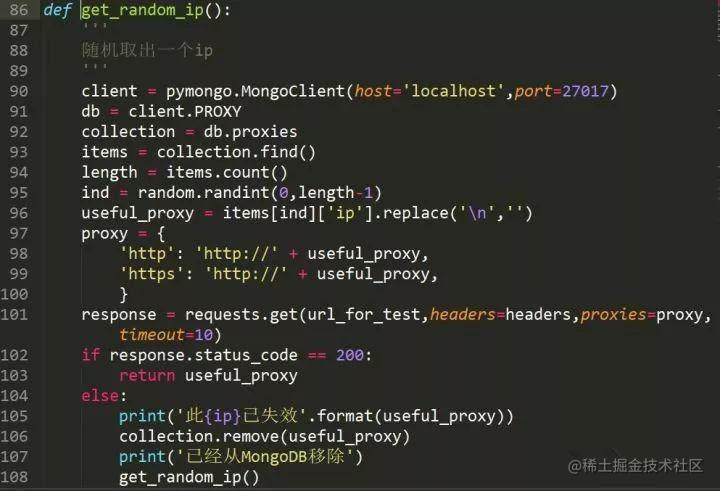``复制代码``
1. `import random`
2. `import requests`
3. `import time`
4. `import pymongo`
5. `from bs4 import BeautifulSoup`
6. ``
7. ``
8. `# 爬取代理的URL地址，选择的是西刺代理`
9. `url_ip = "http://www.xicidaili.com/nt/"`
10. ``
11. `# 设定等待时间`
12. `set_timeout = 5`
13. ``
14. `# 爬取代理的页数，2表示爬取2页的ip地址`
15. `num = 2`
16. ``
17. `# 代理的使用次数`
18. `count_time = 5`
19. ``
20. `# 构造headers`
21. `headers = {'User-Agent': 'Mozilla/5.0 (Windows NT 10.0; WOW64) AppleWebKit/537.36 (KHTML, like Gecko) Chrome/65.0.3325.146 Safari/537.36'}`
22. ``
23. `# 测试ip的URL`
24. `url_for_test = 'http://httpbin.org/ip'`
25. ``
26. ``
27. `def scrawl_xici_ip(num):`
28. `   '''`
29. `   爬取代理ip地址`
30. `   '''  `
31. `   ip_list = []`
32. `   for num_page in range(1,num):`
33. `       url = url_ip + str(num_page)`
34. `       response = requests.get(url,headers=headers)`
35. `       if response.status_code == 200:`
36. `           content = response.text`
37. `           soup = BeautifulSoup(content,'lxml')`
38. `           trs = soup.find_all('tr')`
39. `           for i in range(1,len(trs)):`
40. `               tr = trs[i]`
41. `               tds = tr.find_all('td')      `
42. `               ip_item = tds.text + ':' + tds.text`
43. `               # print(ip_item)`
44. `               ip_list.append(ip_item)`
45. `               ip_set = set(ip_list) # 去掉可能重复的ip`
46. `               ip_list = list(ip_set)`
47. `           time.sleep(count_time) # 等待5秒`
48. `   return ip_list`
49. ``
50. ``
51. `def ip_test(url_for_test,ip_info):`
52. `   '''`
53. `   测试爬取到的ip，测试成功则存入MongoDB`
54. `   '''`
55. `   for ip_for_test in ip_info:`
56. `       # 设置代理`
57. `       proxies = {`
58. `           'http': 'http://' + ip_for_test,`
59. `           'https': 'http://' + ip_for_test,`
60. `           }`
61. `       print(proxies)`
62. `       try:`
63. `           response = requests.get(url_for_test,headers=headers,proxies=proxies,timeout=10)`
64. `           if response.status_code == 200:`
65. `               ip = {'ip':ip_for_test}`
66. `               print(response.text)`
67. `               print('测试通过')`
68. `               write_to_MongoDB(ip)    `
69. `       except Exception as e:`
70. `           print(e)`
71. `           continue`
72. ``
73. ``
74. `def write_to_MongoDB(proxies):`
75. `   '''`
76. `   将测试通过的ip存入MongoDB`
77. `   '''`
78. `   client = pymongo.MongoClient(host='localhost',port=27017)`
79. `   db = client.PROXY`
80. `   collection = db.proxies`
81. `   result = collection.insert(proxies)`
82. `   print(result)`
83. `   print('存储MongoDB成功')`
84. ``
85. ``
86. `def get_random_ip():`
87. `   '''`
88. `   随机取出一个ip`
89. `   '''`
90. `   client = pymongo.MongoClient(host='localhost',port=27017)`
91. `   db = client.PROXY`
92. `   collection = db.proxies`
93. `   items = collection.find()`
94. `   length = items.count()`
95. `   ind = random.randint(0,length-1)`
96. `   useful_proxy = items[ind]['ip'].replace('\n','')`
97. `   proxy = {`
98. `       'http': 'http://' + useful_proxy,`
99. `       'https': 'http://' + useful_proxy,`
100. `       }  `
101. `   response = requests.get(url_for_test,headers=headers,proxies=proxy,timeout=10)`
102. `   if response.status_code == 200:`
103. `       return useful_proxy`
104. `   else:`
105. `       print('此{ip}已失效'.format(useful_proxy))`
106. `       collection.remove(useful_proxy)`
107. `       print('已经从MongoDB移除')`
108. `       get_random_ip()`
109. ``
110. ``
111. `def main():`
112. `   ip_info = []`
113. `   ip_info = scrawl_xici_ip(2)`
114. `   sucess_proxy = ip_test(url_for_test,ip_info)`
115. `   finally_ip = get_random_ip()`
116. `   print('取出的ip为：' + finally_ip)`
117. ``
118. ``
119. ``
120. `if __name__ == '__main__':`
121. `   main()`

\Python中文社区 全球Python中文开发者的 精神部落.jpg")

\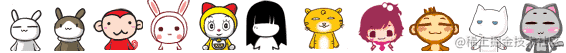\

Python中文社区作为一个去中心化的全球技术社区，以成为全球20万Python中文开发者的精神部落为愿景，目前覆盖各大主流媒体和协作平台，与阿里、腾讯、百度、微软、亚马逊、开源中国、CSDN等业界知名公司和技术社区建立了广泛的联系，拥有来自十多个国家和地区数万名登记会员，会员来自以公安部、工信部、清华大学、北京大学、北京邮电大学、中国人民银行、中科院、中金、华为、BAT、谷歌、微软等为代表的政府机关、科研单位、金融机构以及海内外知名公司，全平台近20万开发者关注。